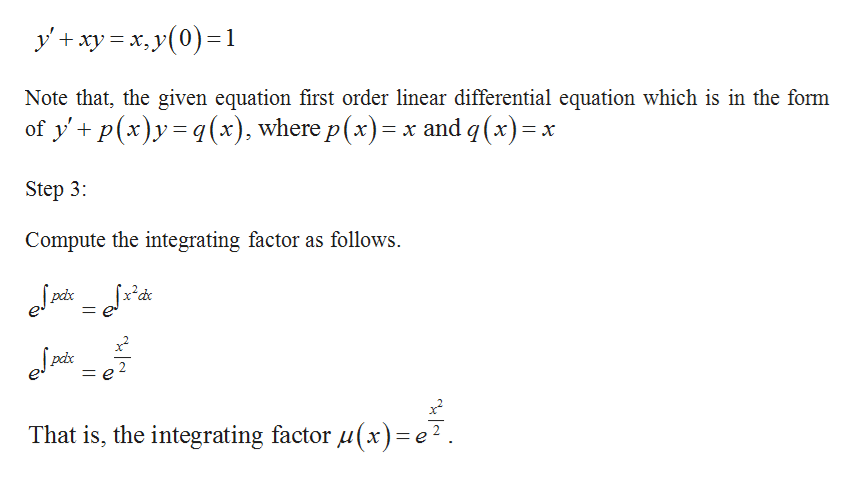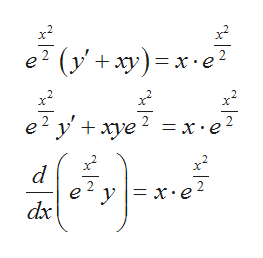Use the method of integrating factors to solve the following initial value problems.y'+xy=x, y(0)=1y'+3x2y= sin(x)e-x^2, y(0)=1

Question

Use the method of integrating factors to solve the following initial value problems.

• y'+xy=x, y(0)=1
• y'+3x2y= sin(x)e-x^2, y(0)=1
Step 1

The given equation are

Step 2

Solve the initial value problemhelp_outlineImage Transcriptioncloseyxyy0)1 Note that, the given equation first order linear differential equation which is in the form of y' p(x)y(x), where p (x) x and q(x) Step 3: Compute the integrating factor as follows That is, the integrating factor ux) = e2. fullscreen
Step 3

Multiply the integrating factor on bot...help_outlineImage Transcriptionclosee? (у'+ ху) — х:е? x2 х e? у+ хуе ? %3Dх-е? 2 d e у 3 х:е dx fullscreen

Want to see the full answer?

See Solution

Want to see this answer and more?

Our solutions are written by experts, many with advanced degrees, and available 24/7

See Solution
Tagged in

Math